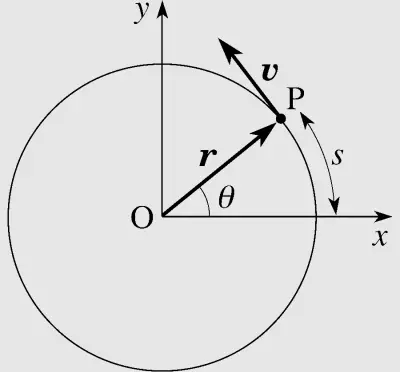# What Is Velocity? Concept, Definition, Formula and TypesVelocity in physics refers to the vector magnitude that indicates the variation of the position of an object for each unit of time. It is a crucial concept for the study of the kinematics of objects.

## What Is Velocity?

In physics we define velocity as a physical magnitude that expresses the degree of displacement of a body with respect to a point in a given time. This variation is expressed in space, therefore it is a vector value, which indicates a magnitude and a direction.There are two types of velocity: average and instantaneous. The first is calculated by dividing the distance covered by the time elapsed, while the instantaneous velocity corresponds to the limit when the time interval tends to zero.

Units in the international system of units are meters per second (m/s), kilometers per hour (km/h), or miles per hour (mph).

## Velocity Types: Linear and Angular

Linear velocity is a derived physical magnitude that is defined as the quotient between the displacement and the time of an object that does not change direction.

However, when a body rotates about an axis, the angular velocity ω appears. The angular velocity indicates the variation of the angular position in time.

Rotating bodies have two types of velocity: angular, which indicates the variation of the angle, and tangential velocity, which is the linear speed with a direction tangent to the curvature at each moment.

## Velocity Formulas

Velocity is defined as the change in the position of an object with respect to time.

### Linear Velocity Formula

The formula for the average velocity of an object is:

v = Δx/Δt

Where:

• v represents velocity.

• Δx represents the change in position or the distance traveled (the final position vector minus the initial position vector)

• Δt represents the elapsed time.

Velocity can be negative, which means that the object is moving backwards. It can also be null, which means that the object is not moving.

### Angular Velocity Formula

To calculate the average angular velocity, the following formula is used:

ω = 2π/T

Where,

• ω is the angular velocity in radians per second

• T is the period of rotation in seconds (the time it takes for the object to complete one revolution)

• π is the constant PI.

## Difference Between Velocity and Speed

Velocity indicates the variation of the position of an object per unit of time. The variation of the position is determined by the difference of two vectors, therefore, velocity is a vector that indicates the direction of the object.

On the other hand, speed refers to the physical magnitude of velocity regardless of direction. The speed tells us the variation of the distance traveled per unit of time but we do not have any information about the direction and position of the object.

## Velocity Examples

Depending on how an object moves or depending on whether it is subjected to acceleration, different types of mo movements are defined:

• In uniform rectilinear motion, the object has constant speed and does not change direction.

• In uniformly accelerated rectilinear motion, the object does not change direction but its speed varies because it is affected by a constant acceleration. In this case, the initial and final speed are different.

• In uniform circular motion, the object rotates on an axis but is not subjected to any acceleration (neither angular nor tangential).

• In uniformly accelerated circular motion, the object rotates on an axis and is subjected to a constant acceleration that can be angular or linear.

Author:

Published: March 16, 2023
Last review: March 16, 2023